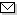Language:   Search:   Contact# Search and Browse the MSC 2000

Search:
Please note that in the second half of 2009, MSC2010 is deployed in the production of ZMATH and will become official standard in January 2010.

Enter a query and click »Search«...

00-XX General → ZMATH
01-XX History and biography [See also the classification number -03 in the other sections] → ZMATH
03-XX Mathematical logic and foundations → ZMATH
05-XX Combinatorics {For finite fields, see 11Txx} → ZMATH
08-XX General algebraic systems → ZMATH
11-XX Number theory → ZMATH
12-XX Field theory and polynomials → ZMATH
13-XX Commutative rings and algebras → ZMATH
14-XX Algebraic geometry → ZMATH
15-XX Linear and multilinear algebra; matrix theory → ZMATH
16-XX Associative rings and algebras {For the commutative case, see 13-XX} → ZMATH
17-XX Nonassociative rings and algebras → ZMATH
18-XX Category theory; homological algebra {For commutative rings see 13Dxx, for associative rings 16Exx, for groups 20Jxx, for topological groups and related structures 57Txx; see also 55Nxx and 55Uxx for algebraic topology} → ZMATH
20-XX Group theory and generalizations → ZMATH
22-XX Topological groups, Lie groups {For transformation groups, see 54H15, 57Sxx, 58-XX. For abstract harmonic analysis, see 43-XX} → ZMATH
28-XX Measure and integration {For analysis on manifolds, see 58-XX} → ZMATH
30-XX Functions of a complex variable {For analysis on manifolds, see 58-XX} → ZMATH
31-XX Potential theory {For probabilistic potential theory, see 60J45} → ZMATH
32-XX Several complex variables and analytic spaces {For infinite-dimensional holomorphy, see 46G20, 58B12} → ZMATH
33-XX Special functions (33-XX deals with the properties of functions as functions) {For orthogonal functions, see 42Cxx; for aspects of combinatorics see 05Axx; for number-theoretic aspects see 11-XX; for representation theory see 22Exx} → ZMATH
34-XX Ordinary differential equations → ZMATH
35-XX Partial differential equations → ZMATH
37-XX Dynamical systems and ergodic theory [See also 26A18, 28Dxx, 34Cxx, 34Dxx, 35Bxx, 46Lxx, 58Jxx, 70-XX] → ZMATH
39-XX Difference and functional equations → ZMATH
40-XX Sequences, series, summability → ZMATH
41-XX Approximations and expansions {For all approximation theory in the complex domain, see 30Exx, 30E05 and 30E10; for all trigonometric approximation and interpolation, see 42Axx, 42A10 and 42A15; for numerical approximation, see 65Dxx} → ZMATH
42-XX Fourier analysis → ZMATH
43-XX Abstract harmonic analysis {For other analysis on topological and Lie groups, see 22Exx} → ZMATH
44-XX Integral transforms, operational calculus {For fractional derivatives and integrals, see 26A33. For Fourier transforms, see 42A38, 42B10. For integral transforms in distribution spaces, see 46F12. For numerical methods, see 65R10} → ZMATH
45-XX Integral equations → ZMATH
46-XX Functional analysis {For manifolds modeled on topological linear spaces, see 57Nxx, 58Bxx} → ZMATH
47-XX Operator theory → ZMATH
49-XX Calculus of variations and optimal control; optimization [See also 34H05, 34K35, 65Kxx, 90Cxx, 93-XX] → ZMATH
51-XX Geometry {For algebraic geometry, see 14-XX} → ZMATH
52-XX Convex and discrete geometry → ZMATH
53-XX Differential geometry {For differential topology, see 57Rxx. For foundational questions of differentiable manifolds, see 58Axx} → ZMATH
54-XX General topology {For the topology of manifolds of all dimensions, see 57Nxx} → ZMATH
55-XX Algebraic topology → ZMATH
57-XX Manifolds and cell complexes {For complex manifolds, see 32Qxx} → ZMATH
58-XX Global analysis, analysis on manifolds {For geometric integration theory, see 49Q15} → ZMATH
60-XX Probability theory and stochastic processes {For additional applications, see 11Kxx, 62-XX, 90-XX, 91-XX, 92-XX, 93-XX, 94-XX} → ZMATH
62-XX Statistics → ZMATH
65-XX Numerical analysis → ZMATH
68-XX Computer science {For papers involving machine computations and programs in a specific mathematical area, see Section -04 in that area} → ZMATH
70-XX Mechanics of particles and systems {For relativistic mechanics, see 83A05 and 83C10; for statistical mechanics, see 82-XX} → ZMATH
74-XX Mechanics of deformable solids → ZMATH
76-XX Fluid mechanics {For general continuum mechanics, see 74Axx, or other parts of 74-XX} → ZMATH
78-XX Optics, electromagnetic theory {For quantum optics, see 81V80} → ZMATH
80-XX Classical thermodynamics, heat transfer {For thermodynamics of solids, see 74A15} → ZMATH
81-XX Quantum theory → ZMATH
82-XX Statistical mechanics, structure of matter → ZMATH
83-XX Relativity and gravitational theory → ZMATH
85-XX Astronomy and astrophysics {For celestial mechanics, see 70F15} → ZMATHAbel prize 2010I. M. Gelfand 1913-2009MSC2010